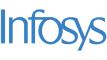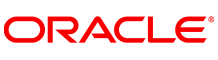New update is available. Click here to update.
Topics

# Binary Search

Easy0/40
Average time to solve is 15m+6 more companies

## Problem statement

You are given an integer array 'A' of size 'N', sorted in non-decreasing order. You are also given an integer 'target'.

Your task is to write a function to search for 'target' in the array 'A'. If it exists, return its index in 0-based indexing. If 'target' is not present in the array 'A', return -1.

Note:

``````You must write an algorithm whose time complexity is O(LogN)
``````

Example:

``````Input: ‘N’ = 7 ‘target’ = 3
‘A’ = [1, 3, 7, 9, 11, 12, 45]

Output: 1

Explanation: A = [1, 3, 7, 9, 11, 12, 45],
The index of element '3' is 1.
``````
Detailed explanation ( Input/output format, Notes, Images )
Constraints :
``````1 <= N <= 10^5
1 <= A[i] <= 10^9
1 <= target <= 10^9
Time Limit: 1 sec
``````
Sample Input 1:
``````7
1 3 7 9 11 12 45
3
``````
Sample Output 1:
``````1
``````
Explanation of sample output 1:
``````nums = [1, 3, 7, 9, 11, 12, 45],
The index of element '3' is 1.
``````

Sample Input 2:
``````7
1 2 3 4 5 6 7
9
``````
Sample Output 2:
``````-1
``````
Explanation of sample output 2:
``````nums = [1, 2, 3, 4, 5, 6, 7],
Element '9' doesn't exist.